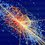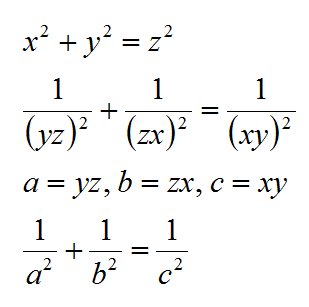# Variation on Pythagorean Theorem

I already know about the Pythagorean Theorem, where $$a^2 + b^2 = c^2$$. Now I'm wondering if there can be a solution where $$\frac {1}{a^2} + \frac {1} {b^2} = \frac {1}{c^2}$$. Is this possible?

If this is impossible tell me how it is impossible to get a solution (or solutions) for this equation.

If this is possible tell me how it is possible to get a solution (or solutions) for this equation.5 years, 7 months ago

This discussion board is a place to discuss our Daily Challenges and the math and science related to those challenges. Explanations are more than just a solution — they should explain the steps and thinking strategies that you used to obtain the solution. Comments should further the discussion of math and science.

When posting on Brilliant:

• Use the emojis to react to an explanation, whether you're congratulating a job well done , or just really confused .
• Ask specific questions about the challenge or the steps in somebody's explanation. Well-posed questions can add a lot to the discussion, but posting "I don't understand!" doesn't help anyone.
• Try to contribute something new to the discussion, whether it is an extension, generalization or other idea related to the challenge.

MarkdownAppears as
*italics* or _italics_ italics
**bold** or __bold__ bold
- bulleted- list
• bulleted
• list
1. numbered2. list
1. numbered
2. list
Note: you must add a full line of space before and after lists for them to show up correctly
paragraph 1paragraph 2

paragraph 1

paragraph 2

[example link](https://brilliant.org)example link
> This is a quote
This is a quote
    # I indented these lines
# 4 spaces, and now they show
# up as a code block.

print "hello world"
# I indented these lines
# 4 spaces, and now they show
# up as a code block.

print "hello world"
MathAppears as
Remember to wrap math in $$ ... $$ or $ ... $ to ensure proper formatting.
2 \times 3 $2 \times 3$
2^{34} $2^{34}$
a_{i-1} $a_{i-1}$
\frac{2}{3} $\frac{2}{3}$
\sqrt{2} $\sqrt{2}$
\sum_{i=1}^3 $\sum_{i=1}^3$
\sin \theta $\sin \theta$
\boxed{123} $\boxed{123}$

Sort by:

We can put it into the form of

$a^2+b^2 = (\frac{ab}{c})^2$

of which one answer is $c = \frac{ab}{\sqrt{a^2+b^2}}$. Some answer of (a, b, c) for natural number $x$ is

$(a, b, c) = (15x, 20x, 12x), (175x, 600x, 168x)$

- 5 years, 6 months ago- 5 years, 6 months ago

for example:

x = 3, y = 4, z = 5

a = 20, b = 15, c = 40

- 5 years, 6 months ago

$a = 20, b =15, c= 12$

- 5 years, 7 months ago

Thank you very much!

- 5 years, 7 months ago

Cool!

- 5 years, 6 months ago